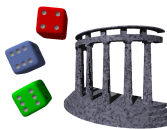# Biblyon the Great

This zine is dedicated to articles about the fantasy role-playing game Gods & Monsters, and other random musings.

Gods & Monsters Fantasy Role-Playing

Beyond here lie dragons

# Random table rolls

Our random table script is doing a lot for us now. We can roll any arbitrary number of times on any text file. But often, we aren’t rolling a known number of times: our number of rolls on the random table is itself random. So it would be nice to, in addition to ./random 3 gems we could also do ./random d4 gems.

Now that we have the dice library installed, we can do this. The main issue is that the script currently knows whether to roll more than once by looking to see if the first item on the command line is all digits:

[toggle code]

• if firstArgument.isdigit():
• count = int(firstArgument)

If we are going to accept die rolls, then we need to accept another character as well as digits: the letter ‘d’. There does, however, have to be at least one number. Basically, we need our script to recognize a die roll.

When we need to recognize text patterns, the most common tool is a regular expression. In Python, we use regular expressions by importing the re library. Add this to the top of the script with the rest of the imports:

• import re

Replace the if/count lines above with:

[toggle code]

• if re.match(r'[0-9]*d?[1-9][0-9]*\$', firstArgument):
• count = firstArgument

That looks complicated—and regular expressions can certainly become complicated—but in this case it isn’t.

1. re.match matches text patterns from the beginning of the text.
2. The last character is a dollar sign, which requires that the pattern match the entire piece of text instead of just the beginning.
3. The first set of characters are in square brackets: [0-9]. Items in square brackets mean, match any character in that range. For example, [a-m] would mean any character from lower-case ‘a’ through lower-case ‘m’. In this case, it’s any number.
4. The next character immediately following the first set is an asterisk. The asterisk means any number of the preceding character. The preceding character is any digit. So, “[0-9]*” means any number of digits. Any number includes zero, so this means zero or more digits at the start of the text.
5. The next portion is the letter ‘d’ followed by a question mark. The letter ‘d’ is needed to show that this is a die roll, and the question mark means that we need zero or one of the previous characters. If this is not a die roll, there will be no ‘d’. If this is a die roll, there will be only one ‘d’. The question mark handles this for us.
6. The next portion is “[1-9]”. There must be at least one digit from 1 to 9 in either a die roll or an arbitrary number.
7. And the final portion is “[0-9]*”. We have already seen this; it means from zero to any amount of digits.

You can construct most of your regular expression search patterns using those rules.

Now that the count is not an integer but can also be a die roll, we need to add some code to the Table.choose method to recognize die rolls. Add the highlighted code:

[toggle code]

• #print some random items from the table
• def choose(self, count):
• if type(count) is not int:
• if count.isdigit():
• count = int(count)
• else:
• count = dice.roll(count + 't')
• if count == 1:
• time = 'time:'
• else:
• time = 'times:'
• print 'Rolling', count, time
• print
• maximumLength = len(unicode(count))
• formatString = '%' + unicode(maximumLength) + 'i. %s'
• for counter in range(count):
• if count > 1:
• print formatString % (counter+1, self.choice())
• else:
• print self.choice()

The new code first checks to see if the count is not an integer; if it isn’t, it checks to see if the variable is all digits; if it is, this is just a number. It turns the text into an integer so that Python can use it for counting, and that’s that.

If the count is not all digits, it must be a die roll. It adds the letter ‘t’ at the end to tell the dice library to total the result, and then rolls that number. The roll result will be an integer. We also print out the result, pluralizing “time” appropriately.

```./random d4 Deep\ Forest
Rolling 2 times:

1. Pixies (17)
2. Apparitions (32)

./random d6 East/gems
Rolling 3 times:

1. jasper
2. chalcedony
3. sapphire
```

In response to Programming for Gamers: Choosing a random item: If you can understand a roleplaying game’s rules, you can understand programming. Programming is a lot easier.